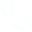# [Information Security] Principle of RSA Asymmetric Encryption Algorithm

Introduction: [Information Security] Principle of RSA asymmetric encryption algorithm (detailed explanation and C++ code implementation)

1. 【Principle of RSA Asymmetric Encryption Algorithm】RSA asymmetric encryption
(1) Select two prime numbers p and q, calculate n=p*q and Euler function φ(n)=(p-1)(q-1), select integer e, make gcd(φ(n), e )=1 (that is, φ(n) and e are co-prime), 1(2) Calculate the inverse of e d=e-1mod φ(n) (ie ed = 1 mod φ(n));
(3) Obtain public key Kpub={e, n}, private key Kpri={d, n} (public public key Kpub, secret private key Kpri);
(4) Encryption (using public key Kpub): for plaintext m(5) Decryption (using private key Kpri): For ciphertext c, plaintext m=cd mod n
2. RSA encryption and decryption example

3. Code implementation (c++)
#include
using namespace std ;

// greatest common factor
int maxCommonDivisor ( int a, int b)
{
int temp = a;
if (a < b)
{
a = b;
b = temp;
}

while (a % b)
{
temp = b;
b = a % b;
a = temp;
}
return b;
}

// least common multiple
int leastCommonMultiple ( int a, int b)
{
int macDivisor = maxCommonDivisor(a, b);
return a / macDivisor * b;
}

// Calculate input ^ rate mod y
int multiMod ( int input, int rate, int y)
{
int start = 1 ;
int arr[ 100 ];
arr[ 0 ] = 1 ;
arr[ 1 ] = input;
int step = 1 ;
int result = 1 ;

while (rate)
{
if (step == 1 )
{
arr[step] = input;
}
else
{
arr[step] = arr[step - 1 ] * arr[step - 1 ];
arr[step] %= y;
}
if (rate& 1 )
{
result *= arr[step];
result %= y;
}
step++;
rate = rate >> 1 ;
}

return result;
}

int main ()
{
int input;
int p, q;
int N, L, E, D;

while ( cin >> p >> q >> input >> E)
{
N = p * q;
// least common multiple
L = leastCommonMultiple(p - 1 , q - 1 );
//E * D mod L = 1
int X = 1 ;
while ((X * L + 1 ) % E)
{
X++;
}

D = (X * L + 1 ) / E;
cout << "N = " << N << " L = " << L << " E = " << E << " D = " << D << " X = " << X << endl ;
// encryption process
int code = multiMod(input, E, N);
// decryption process
int deCode = multiMod(code, D, N);
cout << "code = " << code << " deCode = " << deCode << endl ;
}
}

Copyright statement: The content of this article is contributed by Alibaba Cloud real-name registered users. The copyright belongs to the original author. The Alibaba Cloud developer community does not own the copyright and does not assume the corresponding legal responsibility. For specific rules, please refer to the " Alibaba Cloud Developer Community User Service Agreement " and " Alibaba Cloud Developer Community Intellectual Property Protection Guidelines ". If you find any content suspected of plagiarism in this community, fill out the infringement complaint form to report it. Once verified, this community will delete the allegedly infringing content immediately.

## Related Articles

• ### A detailed explanation of Hadoop core architecture HDFS

Knowledge Base Team

• ### What Does IOT Mean

Knowledge Base Team

• ### 6 Optional Technologies for Data Storage

Knowledge Base Team

• ### What Is Blockchain Technology

Knowledge Base Team

## Explore More Special Offers

1. ### Short Message Service(SMS) & Mail Service

50,000 email package starts as low as USD 1.99, 120 short messages start at only USD 1.00Contact Us